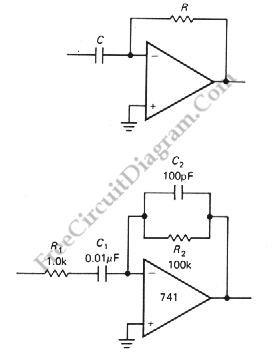# Differentiator Operator with Op-AmpAnother application of OP-AMP is differentiator. Differentiator circuit produce an output if the input is changing. The output can positive or negative, depends on the change of the input: whether it’s decreasing or increasing.  This circuit is a bias stable. The current is produced by the rate of change of input voltage because the inverting input is grounded. Here is the circuit:The current of this circuit is determined by following equation:
I=C*(dVin / dt)
And the ouput voltage is determined by following equation:
Vout=-R*C*(dVin/dt)

The problems of this circuit is instabilities at high frequencies and noise. Those problems is caused by op-amp’s internal phase shift and high gain. The second circuit is used to solve those problems. The roll-off components C2  and R1 depends on the bandwidth of the op-amp and the noise level of the signal. This circuit will change to an integrator at high frequencies because of C2 and R1.